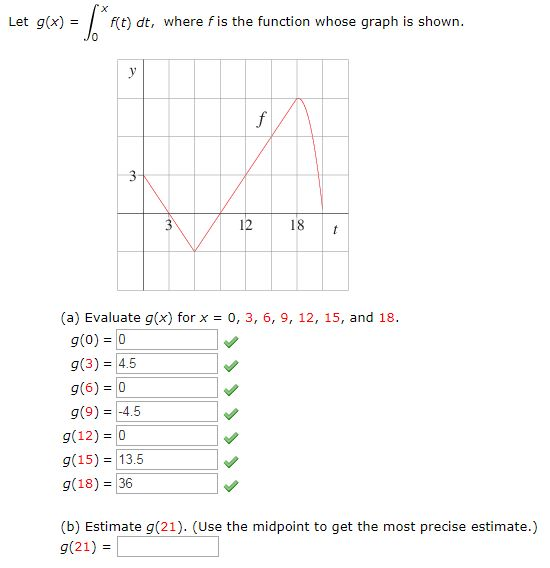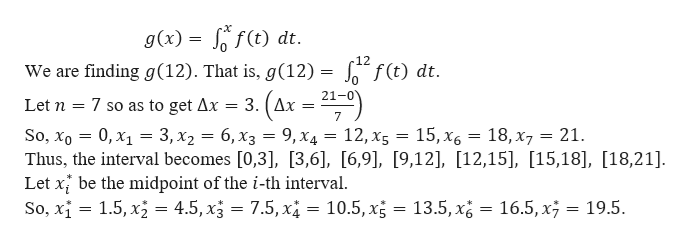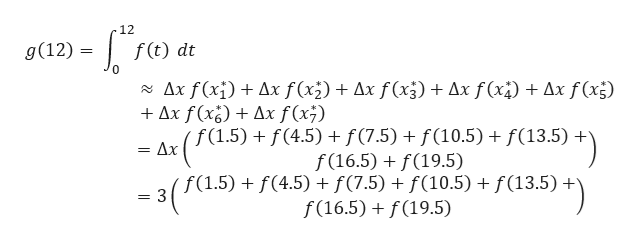# Let g(x)f(t dt, where fis the function whose graph is shown.f3-1812t(a) Evaluate g(x) for x 0, 3 , 6, 9, 12, 15, and 18g(0) 0g(3) 4.5g(6)= 0g(9)4.5g(12) 0g(15) = 13.5g(18) 36(b) Estimate g(21). (Use the midpoint to get the most precise estimate.)g(21)

Question
535 views

What is an estimate of g(21) using the midpoint to get the most precise estimate?help_outlineImage TranscriptioncloseLet g(x) f(t dt, where fis the function whose graph is shown. f 3- 18 12 t (a) Evaluate g(x) for x 0, 3 , 6, 9, 12, 15, and 18 g(0) 0 g(3) 4.5 g(6)= 0 g(9)4.5 g(12) 0 g(15) = 13.5 g(18) 36 (b) Estimate g(21). (Use the midpoint to get the most precise estimate.) g(21) fullscreen
check_circle

Step 1

We need to estimate g(21) by using the mid-point rule.

Note:

By looking at the part (a), it seems that the length of the sub-interval should be 3.

Step 2

We are given thathelp_outlineImage Transcriptioncloseg(x) f(t) dt. 12 We are finding g(12). That is, g(12) = f(t) dt. 21-0 3. (Ax = - Let n 7 so as to get Ax 7 15, Х6 18, x7 21 So, Xo — 0, х1 — 3, х2 — 6, х3 — 9, Хд — 12, х5 Thus, the interval becomes [0,3], [3,6], [6,9], [9,12], [12,15], [15,18], [18,21]. Let x be the midpoint of the i-th interval. So, xi 1.5, x = 4.5, x3 7.5,x4 = 10.5, x5 = 13.5, x = 16.5, x7 19.5. fullscreen
Step 3

Now,

...help_outlineImage Transcriptionclose12 g(12) f(t) dt Ax f(xi)Ax f(x2) Ax f(x5) Ax f(x Ax f(x5) Ax f(x)Ax f(x7) ( f(1.5) + f(4.5) + f(7.5) + f(10.5) + f(13.5) * Δx f(16.5)f(19.5) (f(1.5) + f(4.5) + f(7.5) + f(10.5) f(13.5) * f(16.5)f(19.5) = 3 fullscreen

### Want to see the full answer?

See Solution

#### Want to see this answer and more?

Solutions are written by subject experts who are available 24/7. Questions are typically answered within 1 hour.*

See Solution
*Response times may vary by subject and question.
Tagged in

### Integration